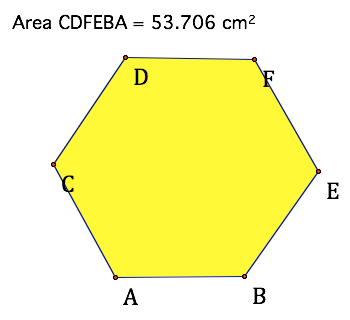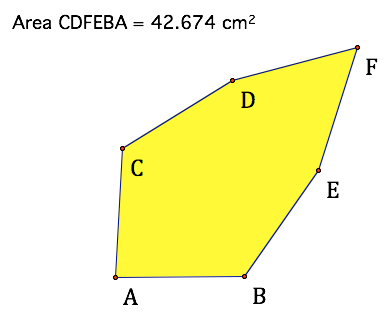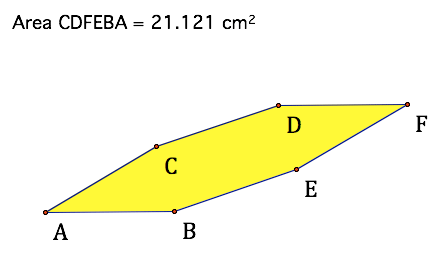SEARCH HOMEMath Central Quandaries & QueriesQuestion from Katie, a student: Hi i have to find the area of a hexagon with only the side length 2cm given would you be able t help?Katie,Perhaps the added assumption is that the hexagon is 'regular' - all angles are the same. If this is the condition, then the interior angles are 120 degrees and there is a unique area. This happens also to be the hexagon with the largest area for the given equal side lengths.

Walter WhiteleyMath Central is supported by the University of Regina and The Pacific Institute for the Mathematical Sciences.